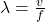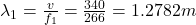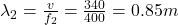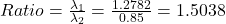## Question 5 An instrument plays a frequency of 266 Hz. Another identical instrument plays a frequency of 400 Hz. How do the wavelength

Question

Question 5
An instrument plays a frequency of 266 Hz. Another identical instrument plays a frequency of 400 Hz. How do the wavelength of each of these
sound waves compare?

in progress 0
2 months 2021-07-25T22:03:15+00:00 1 Answers 2 views 0

the wavelength of the first instrument is approximately 1.5 greater than that produced by the second instrument.

Explanation:

Given:

f₁ = frequency = 266 Hz

f₂ = 400 Hz

Question: How do the wavelength of each of these sound waves compare, λ₁ = ?, λ₂ = ?

The equation to solve this question isHere

λ = wavelength of the sound

v = speed of sound = 340 m/s

f = frequency of each instrument

You need to calculate both wavelengthsAccording to the results, the wavelength of the first instrument is approximately 1.5 greater than that produced by the second instrument.